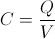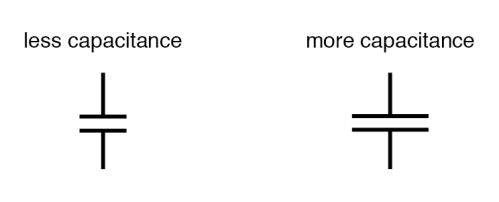What Is A Capacitor? 1
2022-12-22

What Is A Capacitor? 1

Fundamentals

1. Overview

2. Capacitance

3. Dielectric Materials

4. Electric Charge

5. Electric Field

6. Impedance and Reactance

7. Parasitic Inductance

8. Q factor

Capacitors are passive electrical components to store electric energy. In the past they were referred to as condenser. A capacitor is made from electrical conductors that are separated by an insulator. The insulating layer is called a dielectric. Although all capacitors share the same basic principle components, the material choice and configuration can vary widely. They are common elements in electrical circuits. A few examples are to allow only AC current and block DC current, or to smooth a power supply output.

A capacitor is able to store energy in an electrostatic field that is generated by a potential difference across the conductors. So when a conductor is subject to a voltage, one plate of the capacitor will collect positive charge while the other will be negatively charged. The ratio of this electric charge and the potential difference (voltage) is called the capacitance in farads. This is the main parameter to describe a capacitor. The capacitance is the largest when the distance between the conductors is small and the surface of the conductors large. Ideal capacitors are described solely with capacitance, but in the real world some limitations exist. For example, the conductors and lead wires cause parasitic inductance and resistance. The static electric field has a limit on the maximum strength, which is described by the breakdown voltage. The leaking current through the dielectric is called the leakage current.

1. Overview

What is a Capacitor?

A capacitor is a two-terminal passive electrical component that can store electrical energy in an electric field. This effect of a capacitor is known as capacitance. Whilst some capacitance may exists between any two electrical conductors in a circuit, capacitors are components designed to add capacitance to a circuit. The capacitor was originally known as a condenser or condensator but is not widely used nowadays.

Capacitance of a Capacitor

The ability of a conducting body to accumulate charge is known as capacitance. The capacitance value of a capacitor is represented by the formula:where C is the capacitance, Q is the amount of charge stored, and V is the voltage between the two electrodes. One plate equals the amount of charge on the other plate of a capacitor in real life circuits the amount of charge on, but these two charges are of different signs. By examining this formula we can deduce that a 1F (Farad) capacitor holds 1C (Coulomb) of charge when a voltage of 1V (Volt) is applied across its two terminals.

Factors Affecting Capacitance

In constructing a capacitor, there are three basic factors that needs to be determined. All of these factors dictate capacitance by affecting the amount of electric field flux (relative difference of electrons between plates) that will develop for a given amount of electric field force (voltage between the two plates):

Plate Area

All other factors considered equal, greater plate area means greater capacitance; the lesser plate area means lesser capacitance.Plate Spacing

All other factors considered equal, the farther plate spacing gives less capacitance; closer plate spacing gives greater capacitance.